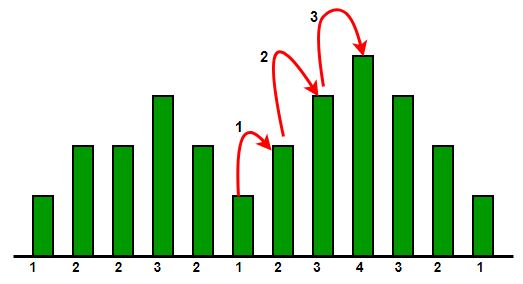# Consecutive steps to roof top

Given heights of consecutive buildings, find the maximum number of consecutive steps one can put forward such that he gain a increase in altitude while going from roof of one building to next adjacent one.

Examples :

```Input : arr[] = {1, 2, 2, 3, 2}
Output : 1
Explanation :
Maximum consecutive steps from 1 to 2 OR  2 to 3.

Input : arr[] = {1, 2, 3, 4}
Output : 3
```

## Recommended: Please solve it on “PRACTICE” first, before moving on to the solution.This problem is basically a variation of Longest increasing subarray

Approach:-

```initialize count = 0
initialize maximum = 0
if arr[i]>a[i-1]
then count increment
else
maximum = max(maximum, count)

at the end maximum=max(maximum, count)
```

## C++

 `// CPP code to find maximum  ` `// number of consecutive steps. ` `#include ` `using` `namespace` `std; ` ` `  `// Function to count consecutive steps ` `int` `find_consecutive_steps(``int` `arr[], ``int` `len) ` `{ ` `    ``int` `count = 0; ` `    ``int` `maximum = 0; ` ` `  `    ``for` `(``int` `index = 1; index < len; index++) { ` `         `  `        ``// count the number of consecutive  ` `        ``// increasing height building ` `        ``if` `(arr[index] > arr[index - 1]) ` `            ``count++; ` `        ``else` `        ``{ ` `            ``maximum = max(maximum, count); ` `            ``count = 0; ` `        ``} ` `    ``} ` `     `  `    ``return` `max(maximum, count);     ` `} ` ` `  `// Driver code ` `int` `main() ` `{ ` `    ``int` `arr[] = { 1, 2, 3, 4 }; ` `    ``int` `len = ``sizeof``(arr) / ``sizeof``(arr); ` ` `  `    ``cout << find_consecutive_steps(arr, len); ` `} `

## Java

 `// Java code to find maximum  ` `// number of consecutive steps. ` `import` `java.io.*; ` ` `  `class` `GFG { ` `     `  `    ``// Function to count consecutive steps ` `    ``static` `int` `find_consecutive_steps(``int` `arr[], ` `                                        ``int` `len) ` `    ``{ ` `        ``int` `count = ``0``; ` `        ``int` `maximum = ``0``; ` `     `  `        ``for` `(``int` `index = ``1``; index < len; index++) { ` `             `  `            ``// count the number of consecutive  ` `            ``// increasing height building ` `            ``if` `(arr[index] > arr[index - ``1``]) ` `                ``count++; ` `            ``else` `            ``{ ` `                ``maximum = Math.max(maximum, count); ` `                ``count = ``0``; ` `            ``} ` `        ``} ` `         `  `        ``return` `Math.max(maximum, count);  ` `    ``} ` `     `  `    ``// Driver code ` `    ``public` `static` `void` `main (String[] args) { ` `         `  `        ``int` `arr[] = { ``1``, ``2``, ``3``, ``4` `}; ` `        ``int` `len = arr.length; ` `      `  `        ``System.out.println(find_consecutive_steps(arr, ` `                                                ``len)); ` `    ``} ` `} ` ` `  `// This code is contributed by Gitanjali. `

## Python3

 `# Python3 code to find maximum  ` `# number of consecutive steps ` `import` `math ` ` `  `# Function to count consecutive steps ` `def` `find_consecutive_steps(arr, ``len``): ` ` `  `    ``count ``=` `0``; maximum ``=` `0` ` `  `    ``for` `index ``in` `range``(``1``, ``len``): ` `         `  `        ``# count the number of consecutive  ` `        ``# increasing height building ` `        ``if` `(arr[index] > arr[index ``-` `1``]): ` `            ``count ``+``=` `1` `             `  `        ``else``: ` `            ``maximum ``=` `max``(maximum, count) ` `            ``count ``=` `0` `     `  `    ``return` `max``(maximum, count) ` ` `  `# Driver code ` `arr ``=` `[ ``1``, ``2``, ``3``, ``4` `] ` `len` `=` `len``(arr) ` `print``(find_consecutive_steps(arr, ``len``)) ` ` `  ` `  `# This code is contributed by Gitanjali. `

## C#

 `// C# code to find maximum  ` `// number of consecutive steps. ` `using` `System; ` ` `  `class` `GFG { ` `     `  `    ``// Function to count consecutive steps ` `    ``static` `int` `find_consecutive_steps(``int` `[]arr, ` `                                        ``int` `len) ` `    ``{ ` `        ``int` `count = 0; ` `        ``int` `maximum = 0; ` `     `  `        ``for` `(``int` `index = 1; index < len; index++) { ` `             `  `            ``// count the number of consecutive  ` `            ``// increasing height building ` `            ``if` `(arr[index] > arr[index - 1]) ` `                ``count++; ` `            ``else` `            ``{ ` `                ``maximum = Math.Max(maximum, count); ` `                ``count = 0; ` `            ``} ` `        ``} ` `         `  `        ``return` `Math.Max(maximum, count);  ` `    ``} ` `     `  `    ``// Driver code ` `    ``public` `static` `void` `Main () { ` `         `  `        ``int` `[]arr = { 1, 2, 3, 4 }; ` `        ``int` `len = arr.Length; ` `     `  `        ``Console.WriteLine(find_consecutive_steps(arr, ` `                                                ``len)); ` `    ``} ` `} ` ` `  `// This code is contributed by vt_m. `

## PHP

 ` ``\$arr``[``\$index` `- 1]) ` `            ``\$count``++; ` `        ``else` `        ``{ ` `            ``\$maximum` `= max(``\$maximum``, ``\$count``); ` `            ``\$count` `= 0; ` `        ``} ` `    ``} ` `     `  `    ``return` `max(``\$maximum``, ``\$count``);  ` `} ` ` `  `// Driver code ` `\$arr` `= ``array``( 1, 2, 3, 4 ); ` `\$len` `= ``count``(``\$arr``); ` ` `  `echo` `find_consecutive_steps(``\$arr``, ``\$len``); ` ` `  `// This code is contributed by vt_m.  ` `?> `

Output :

```3
```

Attention reader! Don’t stop learning now. Get hold of all the important DSA concepts with the DSA Self Paced Course at a student-friendly price and become industry ready.

My Personal Notes arrow_drop_upCheck out this Author's contributed articles.

If you like GeeksforGeeks and would like to contribute, you can also write an article using contribute.geeksforgeeks.org or mail your article to contribute@geeksforgeeks.org. See your article appearing on the GeeksforGeeks main page and help other Geeks.

Please Improve this article if you find anything incorrect by clicking on the "Improve Article" button below.

Improved By : vt_m

Article Tags :
Practice Tags :

1

Please write to us at contribute@geeksforgeeks.org to report any issue with the above content.